## 5.1 One numerical explanatory variable

Why do some professors and instructors at universities and colleges receive high teaching evaluations scores from students while others receive lower ones? Are there differences in teaching evaluations between instructors of different demographic groups? Could there be an impact due to student biases? These are all questions that are of interest to university/college administrators, as teaching evaluations are among the many criteria considered in determining which instructors and professors get promoted.

Researchers at the University of Texas in Austin, Texas (UT Austin) tried to answer the following research question: what factors explain differences in instructor teaching evaluation scores? To this end, they collected instructor and course information on 463 courses. A full description of the study can be found at openintro.org.

In this section, we’ll keep things simple for now and try to explain differences in instructor teaching scores as a function of one numerical variable: the instructor’s “beauty” score (we’ll describe how this score was determined shortly). Could it be that instructors with higher “beauty” scores also have higher teaching evaluations? Could it be instead that instructors with higher “beauty” scores tend to have lower teaching evaluations? Or could it be that there is no relationship between “beauty” score and teaching evaluations? We’ll answer these questions by modeling the relationship between teaching scores and “beauty” scores using simple linear regression where we have:

1. A numerical outcome variable $$y$$ (the instructor’s teaching score) and
2. A single numerical explanatory variable $$x$$ (the instructor’s “beauty” score).

### 5.1.1 Exploratory data analysis

The data on the 463 courses at UT Austin can be found in the evals data frame included in the moderndive package. However, to keep things simple, let’s select() only the subset of the variables we’ll consider in this chapter, and save this data in a new data frame called evals_ch5:

evals_ch5 <- evals %>%
select(ID, score, bty_avg, age)

A crucial step before doing any kind of analysis or modeling is performing an exploratory data analysis, or EDA for short. EDA gives you a sense of the distributions of the individual variables in your data, whether any potential relationships exist between variables, whether there are outliers and/or missing values, and (most importantly) how to build your model. Here are three common steps in an EDA:

1. Most crucially, looking at the raw data values.
2. Computing summary statistics, such as means, medians, and interquartile ranges.
3. Creating data visualizations.

Let’s perform the first common step in an exploratory data analysis: looking at the raw data values. Because this step seems so trivial, unfortunately many data analysts ignore it. However, getting an early sense of what your raw data looks like can often prevent many larger issues down the road.

You can do this by using RStudio’s spreadsheet viewer or by using the glimpse() function as introduced in Subsection 1.4.3 on exploring data frames:

glimpse(evals_ch5)
Rows: 463
Columns: 4
$ID <int> 1, 2, 3, 4, 5, 6, 7, 8, 9, 10, 11, 12, 13, 14, 15, 16, 17, 18…$ score   <dbl> 4.7, 4.1, 3.9, 4.8, 4.6, 4.3, 2.8, 4.1, 3.4, 4.5, 3.8, 4.5, 4…
$bty_avg <dbl> 5.00, 5.00, 5.00, 5.00, 3.00, 3.00, 3.00, 3.33, 3.33, 3.17, 3…$ age     <int> 36, 36, 36, 36, 59, 59, 59, 51, 51, 40, 40, 40, 40, 40, 40, 4…

Observe that Observations: 463 indicates that there are 463 rows/observations in evals_ch5, where each row corresponds to one observed course at UT Austin. It is important to note that the observational unit is an individual course and not an individual instructor. Recall from Subsection 1.4.3 that the observational unit is the “type of thing” that is being measured by our variables. Since instructors teach more than one course in an academic year, the same instructor will appear more than once in the data. Hence there are fewer than 463 unique instructors being represented in evals_ch5. We’ll revisit this idea in Section 10.3, when we talk about the “independence assumption” for inference for regression.

A full description of all the variables included in evals can be found at openintro.org or by reading the associated help file (run ?evals in the console). However, let’s fully describe only the 4 variables we selected in evals_ch5:

1. ID: An identification variable used to distinguish between the 1 through 463 courses in the dataset.
2. score: A numerical variable of the course instructor’s average teaching score, where the average is computed from the evaluation scores from all students in that course. Teaching scores of 1 are lowest and 5 are highest. This is the outcome variable $$y$$ of interest.
3. bty_avg: A numerical variable of the course instructor’s average “beauty” score, where the average is computed from a separate panel of six students. “Beauty” scores of 1 are lowest and 10 are highest. This is the explanatory variable $$x$$ of interest.
4. age: A numerical variable of the course instructor’s age. This will be another explanatory variable $$x$$ that we’ll use in the Learning check at the end of this subsection.

An alternative way to look at the raw data values is by choosing a random sample of the rows in evals_ch5 by piping it into the sample_n() function from the dplyr package. Here we set the size argument to be 5, indicating that we want a random sample of 5 rows. We display the results in Table 5.1. Note that due to the random nature of the sampling, you will likely end up with a different subset of 5 rows.

evals_ch5 %>%
sample_n(size = 5)
TABLE 5.1: A random sample of 5 out of the 463 courses at UT Austin
ID score bty_avg age
129 3.7 3.00 62
109 4.7 4.33 46
28 4.8 5.50 62
434 2.8 2.00 62
330 4.0 2.33 64

Now that we’ve looked at the raw values in our evals_ch5 data frame and got a preliminary sense of the data, let’s move on to the next common step in an exploratory data analysis: computing summary statistics. Let’s start by computing the mean and median of our numerical outcome variable score and our numerical explanatory variable “beauty” score denoted as bty_avg. We’ll do this by using the summarize() function from dplyr along with the mean() and median() summary functions we saw in Section 3.3.

evals_ch5 %>%
summarize(mean_bty_avg = mean(bty_avg), mean_score = mean(score),
median_bty_avg = median(bty_avg), median_score = median(score))
# A tibble: 1 x 4
mean_bty_avg mean_score median_bty_avg median_score
<dbl>      <dbl>          <dbl>        <dbl>
1         4.42       4.17           4.33          4.3

However, what if we want other summary statistics as well, such as the standard deviation (a measure of spread), the minimum and maximum values, and various percentiles?

Typing out all these summary statistic functions in summarize() would be long and tedious. Instead, let’s use the convenient skim() function from the skimr package. This function takes in a data frame, “skims” it, and returns commonly used summary statistics. Let’s take our evals_ch5 data frame, select() only the outcome and explanatory variables teaching score and bty_avg, and pipe them into the skim() function:

evals_ch5 %>% select(score, bty_avg) %>% skim()
Skim summary statistics
n obs: 463
n variables: 2

── Variable type:numeric
variable missing complete   n mean   sd   p0  p25  p50 p75 p100
bty_avg       0      463 463 4.42 1.53 1.67 3.17 4.33 5.5 8.17
score       0      463 463 4.17 0.54 2.3  3.8  4.3  4.6 5   

(For formatting purposes in this book, the inline histogram that is usually printed with skim() has been removed. This can be done by using skim_with(numeric = list(hist = NULL)) prior to using the skim() function for version 1.0.6 of skimr.)

For the numerical variables teaching score and bty_avg it returns:

• missing: the number of missing values
• complete: the number of non-missing or complete values
• n: the total number of values
• mean: the average
• sd: the standard deviation
• p0: the 0th percentile: the value at which 0% of observations are smaller than it (the minimum value)
• p25: the 25th percentile: the value at which 25% of observations are smaller than it (the 1st quartile)
• p50: the 50th percentile: the value at which 50% of observations are smaller than it (the 2nd quartile and more commonly called the median)
• p75: the 75th percentile: the value at which 75% of observations are smaller than it (the 3rd quartile)
• p100: the 100th percentile: the value at which 100% of observations are smaller than it (the maximum value)

Looking at this output, we can see how the values of both variables distribute. For example, the mean teaching score was 4.17 out of 5, whereas the mean “beauty” score was 4.42 out of 10. Furthermore, the middle 50% of teaching scores was between 3.80 and 4.6 (the first and third quartiles), whereas the middle 50% of “beauty” scores falls within 3.17 to 5.5 out of 10.

The skim() function only returns what are known as univariate summary statistics: functions that take a single variable and return some numerical summary of that variable. However, there also exist bivariate summary statistics: functions that take in two variables and return some summary of those two variables. In particular, when the two variables are numerical, we can compute the correlation coefficient. Generally speaking, coefficients are quantitative expressions of a specific phenomenon. A correlation coefficient is a quantitative expression of the strength of the linear relationship between two numerical variables. Its value ranges between -1 and 1 where:

• -1 indicates a perfect negative relationship: As one variable increases, the value of the other variable tends to go down, following a straight line.
• 0 indicates no relationship: The values of both variables go up/down independently of each other.
• +1 indicates a perfect positive relationship: As the value of one variable goes up, the value of the other variable tends to go up as well in a linear fashion.

Figure 5.1 gives examples of 9 different correlation coefficient values for hypothetical numerical variables $$x$$ and $$y$$. For example, observe in the top right plot that for a correlation coefficient of -0.75 there is a negative linear relationship between $$x$$ and $$y$$, but it is not as strong as the negative linear relationship between $$x$$ and $$y$$ when the correlation coefficient is -0.9 or -1.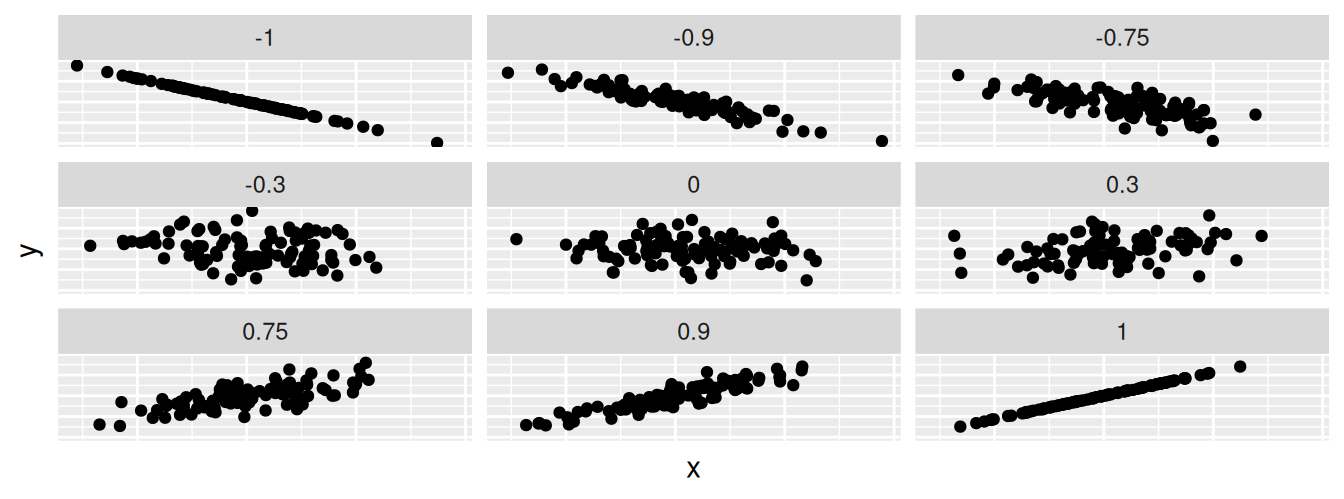FIGURE 5.1: Nine different correlation coefficients.

The correlation coefficient can be computed using the get_correlation() function in the moderndive package. In this case, the inputs to the function are the two numerical variables for which we want to calculate the correlation coefficient.

We put the name of the outcome variable on the left-hand side of the ~ “tilde” sign, while putting the name of the explanatory variable on the right-hand side. This is known as R’s formula notation. We will use this same “formula” syntax with regression later in this chapter.

evals_ch5 %>%
get_correlation(formula = score ~ bty_avg)
# A tibble: 1 x 1
cor
<dbl>
1 0.187

An alternative way to compute correlation is to use the cor() summary function within a summarize():

evals_ch5 %>%
summarize(correlation = cor(score, bty_avg))

In our case, the correlation coefficient of 0.187 indicates that the relationship between teaching evaluation score and “beauty” average is “weakly positive.” There is a certain amount of subjectivity in interpreting correlation coefficients, especially those that aren’t close to the extreme values of -1, 0, and 1. To develop your intuition about correlation coefficients, play the “Guess the Correlation” 1980’s style video game mentioned in Subsection 5.4.1.

Let’s now perform the last of the steps in an exploratory data analysis: creating data visualizations. Since both the score and bty_avg variables are numerical, a scatterplot is an appropriate graph to visualize this data. Let’s do this using geom_point() and display the result in Figure 5.2. Furthermore, let’s highlight the six points in the top right of the visualization in a box.

ggplot(evals_ch5, aes(x = bty_avg, y = score)) +
geom_point() +
labs(x = "Beauty Score",
y = "Teaching Score",
title = "Scatterplot of relationship of teaching and beauty scores")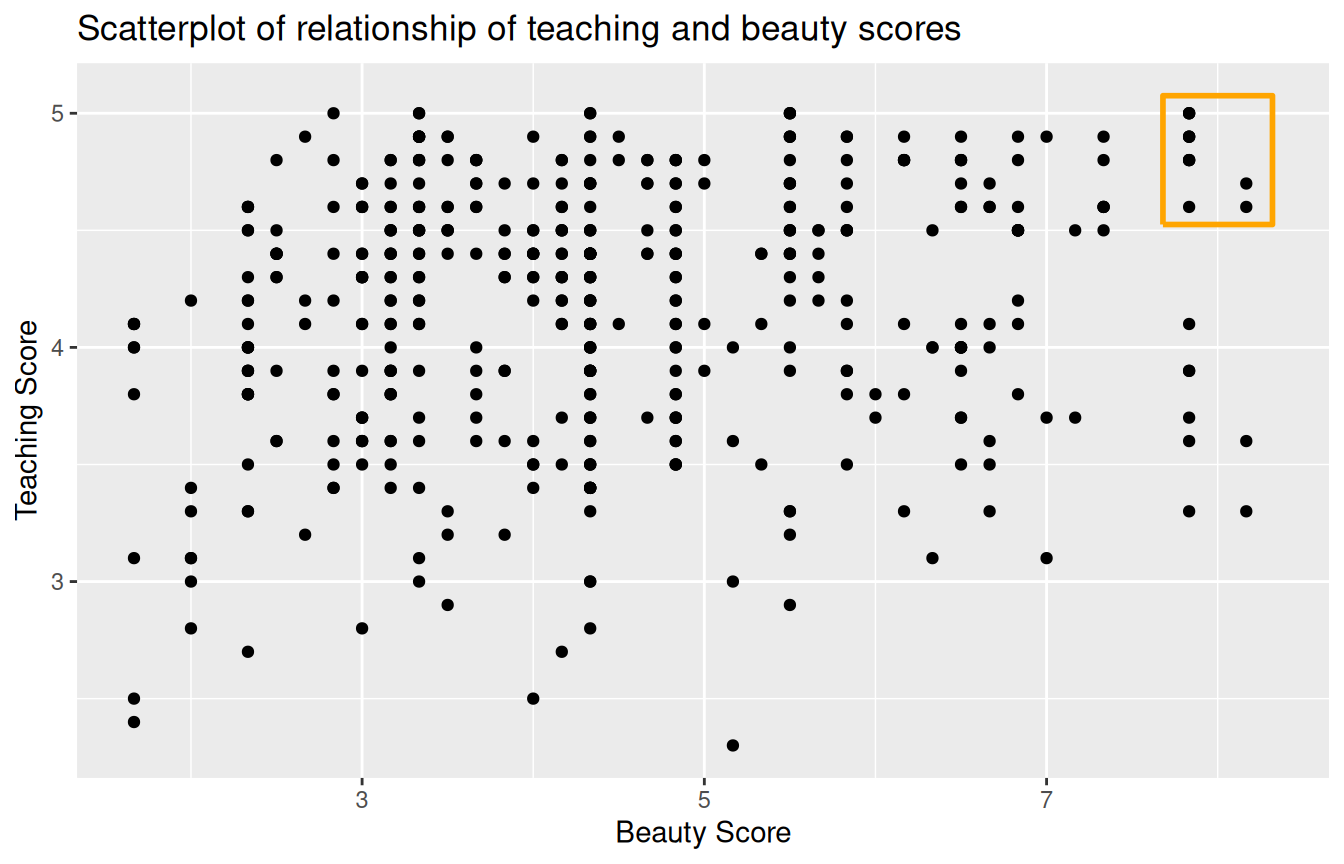FIGURE 5.2: Instructor evaluation scores at UT Austin.

Observe that most “beauty” scores lie between 2 and 8, while most teaching scores lie between 3 and 5. Furthermore, while opinions may vary, it is our opinion that the relationship between teaching score and “beauty” score is “weakly positive.” This is consistent with our earlier computed correlation coefficient of 0.187.

Furthermore, there appear to be six points in the top-right of this plot highlighted in the box. However, this is not actually the case, as this plot suffers from overplotting. Recall from Subsection 2.3.2 that overplotting occurs when several points are stacked directly on top of each other, making it difficult to distinguish them. So while it may appear that there are only six points in the box, there are actually more. This fact is only apparent when using geom_jitter() in place of geom_point(). We display the resulting plot in Figure 5.3 along with the same small box as in Figure 5.2.

ggplot(evals_ch5, aes(x = bty_avg, y = score)) +
geom_jitter() +
labs(x = "Beauty Score", y = "Teaching Score",
title = "Scatterplot of relationship of teaching and beauty scores")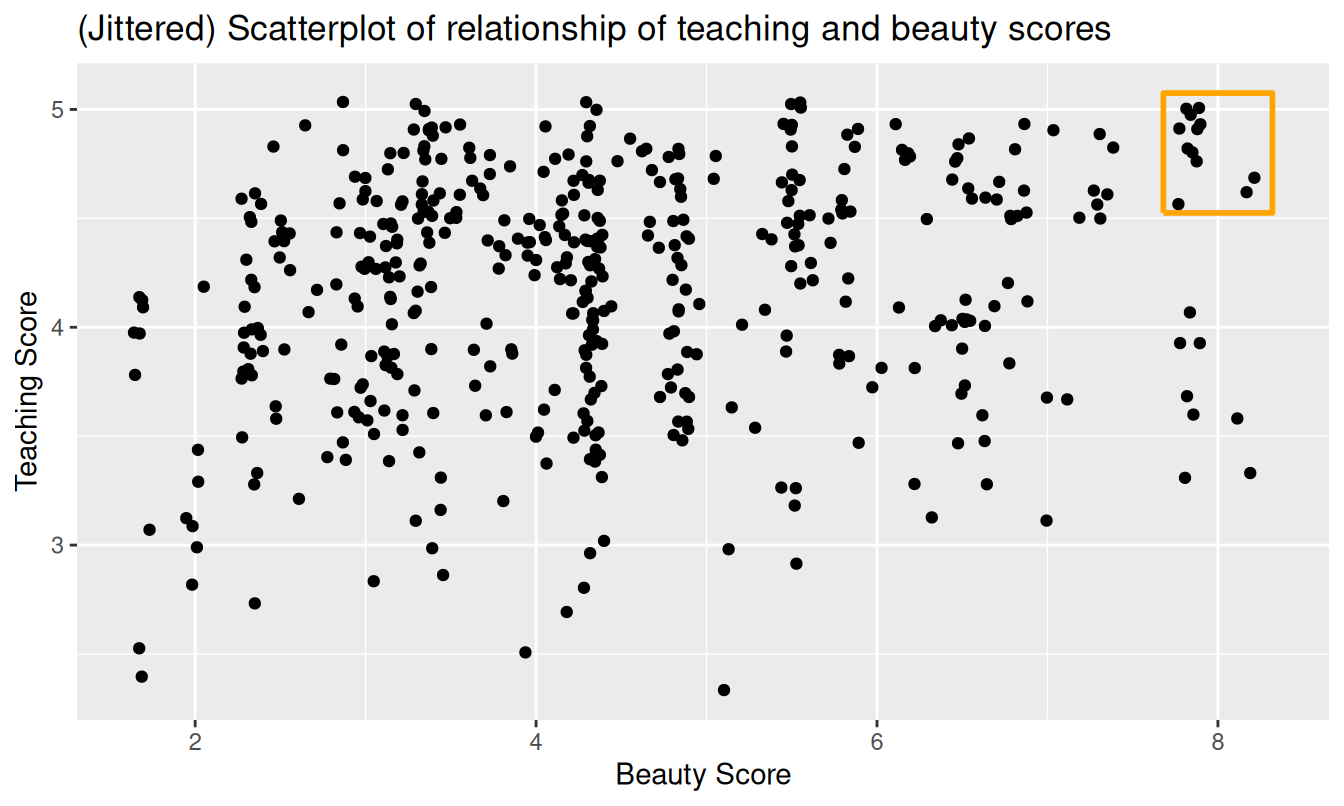FIGURE 5.3: Instructor evaluation scores at UT Austin.

It is now apparent that there are 12 points in the area highlighted in the box and not six as originally suggested in Figure 5.2. Recall from Subsection 2.3.2 on overplotting that jittering adds a little random “nudge” to each of the points to break up these ties. Furthermore, recall that jittering is strictly a visualization tool; it does not alter the original values in the data frame evals_ch5. To keep things simple going forward, however, we’ll only present regular scatterplots rather than their jittered counterparts.

Let’s build on the unjittered scatterplot in Figure 5.2 by adding a “best-fitting” line: of all possible lines we can draw on this scatterplot, it is the line that “best” fits through the cloud of points. We do this by adding a new geom_smooth(method = "lm", se = FALSE) layer to the ggplot() code that created the scatterplot in Figure 5.2. The method = "lm" argument sets the line to be a “linear model.” The se = FALSE argument suppresses standard error uncertainty bars. (We’ll define the concept of standard error later in Subsection 7.3.2.)

ggplot(evals_ch5, aes(x = bty_avg, y = score)) +
geom_point() +
labs(x = "Beauty Score", y = "Teaching Score",
title = "Relationship between teaching and beauty scores") +
geom_smooth(method = "lm", se = FALSE)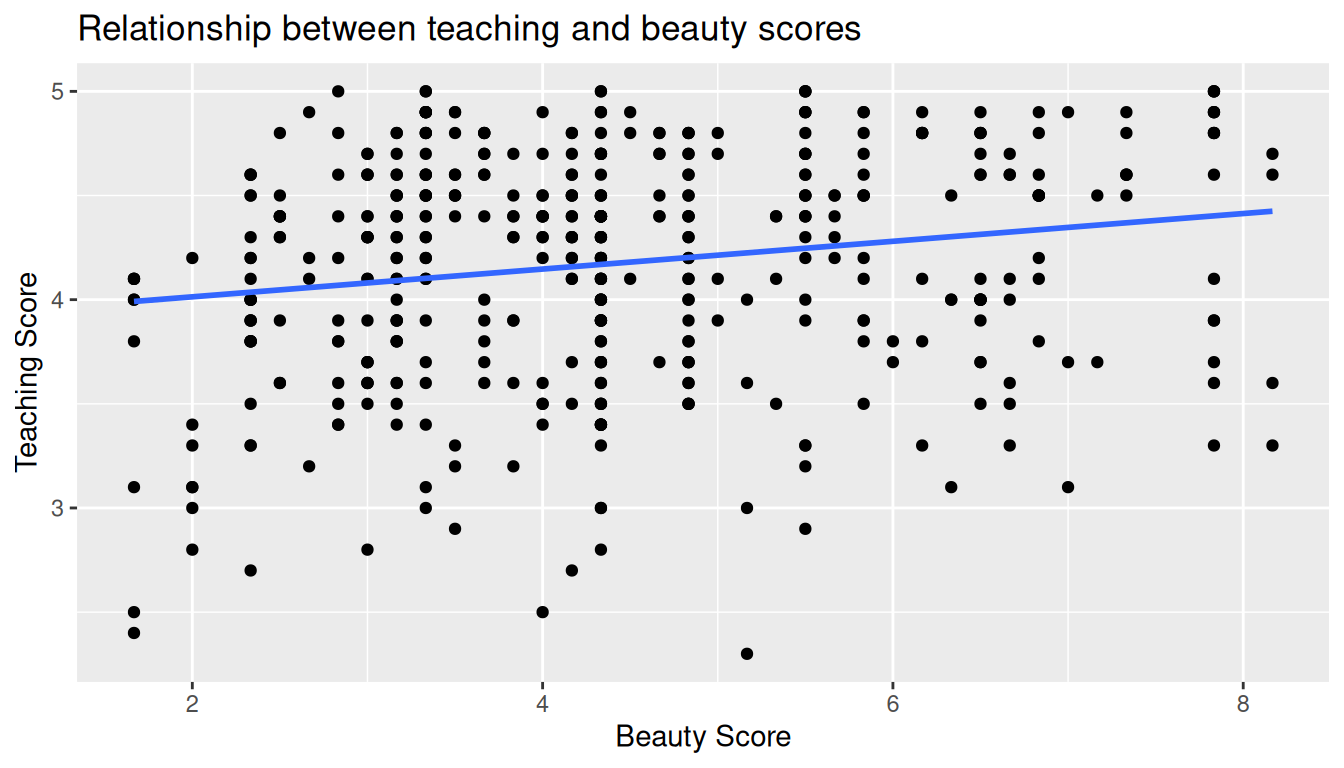FIGURE 5.4: Regression line.

The line in the resulting Figure 5.4 is called a “regression line.” The regression line is a visual summary of the relationship between two numerical variables, in our case the outcome variable score and the explanatory variable bty_avg. The positive slope of the blue line is consistent with our earlier observed correlation coefficient of 0.187 suggesting that there is a positive relationship between these two variables: as instructors have higher “beauty” scores, so also do they receive higher teaching evaluations. We’ll see later, however, that while the correlation coefficient and the slope of a regression line always have the same sign (positive or negative), they typically do not have the same value.

Furthermore, a regression line is “best-fitting” in that it minimizes some mathematical criteria. We present these mathematical criteria in Subsection 5.3.2, but we suggest you read this subsection only after first reading the rest of this section on regression with one numerical explanatory variable.

Learning check

(LC5.1) Conduct a new exploratory data analysis with the same outcome variable $$y$$ being score but with age as the new explanatory variable $$x$$. Remember, this involves three things:

1. Looking at the raw data values.
2. Computing summary statistics.
3. Creating data visualizations.

What can you say about the relationship between age and teaching scores based on this exploration?

### 5.1.2 Simple linear regression

You may recall from secondary/high school algebra that the equation of a line is $$y = a + b\cdot x$$. (Note that the $$\cdot$$ symbol is equivalent to the $$\times$$ “multiply by” mathematical symbol. We’ll use the $$\cdot$$ symbol in the rest of this book as it is more succinct.) It is defined by two coefficients $$a$$ and $$b$$. The intercept coefficient $$a$$ is the value of $$y$$ when $$x = 0$$. The slope coefficient $$b$$ for $$x$$ is the increase in $$y$$ for every increase of one in $$x$$. This is also called the “rise over run.”

However, when defining a regression line like the regression line in Figure 5.4, we use slightly different notation: the equation of the regression line is $$\widehat{y} = b_0 + b_1 \cdot x$$ . The intercept coefficient is $$b_0$$, so $$b_0$$ is the value of $$\widehat{y}$$ when $$x = 0$$. The slope coefficient for $$x$$ is $$b_1$$, i.e., the increase in $$\widehat{y}$$ for every increase of one in $$x$$. Why do we put a “hat” on top of the $$y$$? It’s a form of notation commonly used in regression to indicate that we have a “fitted value,” or the value of $$y$$ on the regression line for a given $$x$$ value. We’ll discuss this more in the upcoming Subsection 5.1.3.

We know that the regression line in Figure 5.4 has a positive slope $$b_1$$ corresponding to our explanatory $$x$$ variable bty_avg. Why? Because as instructors tend to have higher bty_avg scores, so also do they tend to have higher teaching evaluation scores. However, what is the numerical value of the slope $$b_1$$? What about the intercept $$b_0$$? Let’s not compute these two values by hand, but rather let’s use a computer!

We can obtain the values of the intercept $$b_0$$ and the slope for bty_avg $$b_1$$ by outputting a linear regression table. This is done in two steps:

1. We first “fit” the linear regression model using the lm() function and save it in score_model.
2. We get the regression table by applying the get_regression_table() function from the moderndive package to score_model.
# Fit regression model:
score_model <- lm(score ~ bty_avg, data = evals_ch5)
# Get regression table:
get_regression_table(score_model)
TABLE 5.2: Linear regression table
term estimate std_error statistic p_value lower_ci upper_ci
intercept 3.880 0.076 50.96 0 3.731 4.030
bty_avg 0.067 0.016 4.09 0 0.035 0.099

Let’s first focus on interpreting the regression table output in Table 5.2, and then we’ll later revisit the code that produced it. In the estimate column of Table 5.2 are the intercept $$b_0$$ = 3.88 and the slope $$b_1$$ = 0.067 for bty_avg. Thus the equation of the regression line in Figure 5.4 follows:

\begin{aligned} \widehat{y} &= b_0 + b_1 \cdot x\\ \widehat{\text{score}} &= b_0 + b_{\text{bty}\_\text{avg}} \cdot\text{bty}\_\text{avg}\\ &= 3.88 + 0.067\cdot\text{bty}\_\text{avg} \end{aligned}

The intercept $$b_0$$ = 3.88 is the average teaching score $$\widehat{y}$$ = $$\widehat{\text{score}}$$ for those courses where the instructor had a “beauty” score bty_avg of 0. Or in graphical terms, it’s where the line intersects the $$y$$ axis when $$x$$ = 0. Note, however, that while the intercept of the regression line has a mathematical interpretation, it has no practical interpretation here, since observing a bty_avg of 0 is impossible; it is the average of six panelists’ “beauty” scores ranging from 1 to 10. Furthermore, looking at the scatterplot with the regression line in Figure 5.4, no instructors had a “beauty” score anywhere near 0.

Of greater interest is the slope $$b_1$$ = $$b_{\text{bty}\_\text{avg}}$$ for bty_avg of 0.067, as this summarizes the relationship between the teaching and “beauty” score variables. Note that the sign is positive, suggesting a positive relationship between these two variables, meaning teachers with higher “beauty” scores also tend to have higher teaching scores. Recall from earlier that the correlation coefficient is 0.187. They both have the same positive sign, but have a different value. Recall further that the correlation’s interpretation is the “strength of linear association”. The slope’s interpretation is a little different:

For every increase of 1 unit in bty_avg, there is an associated increase of, on average, 0.067 units of score.

We only state that there is an associated increase and not necessarily a causal increase. For example, perhaps it’s not that higher “beauty” scores directly cause higher teaching scores per se. Instead, the following could hold true: individuals from wealthier backgrounds tend to have stronger educational backgrounds and hence have higher teaching scores, while at the same time these wealthy individuals also tend to have higher “beauty” scores. In other words, just because two variables are strongly associated, it doesn’t necessarily mean that one causes the other. This is summed up in the often quoted phrase, “correlation is not necessarily causation.” We discuss this idea further in Subsection 5.3.1.

Furthermore, we say that this associated increase is on average 0.067 units of teaching score, because you might have two instructors whose bty_avg scores differ by 1 unit, but their difference in teaching scores won’t necessarily be exactly 0.067. What the slope of 0.067 is saying is that across all possible courses, the average difference in teaching score between two instructors whose “beauty” scores differ by one is 0.067.

Now that we’ve learned how to compute the equation for the regression line in Figure 5.4 using the values in the estimate column of Table 5.2, and how to interpret the resulting intercept and slope, let’s revisit the code that generated this table:

# Fit regression model:
score_model <- lm(score ~ bty_avg, data = evals_ch5)
# Get regression table:
get_regression_table(score_model)

First, we “fit” the linear regression model to the data using the lm() function and save this as score_model. When we say “fit”, we mean “find the best fitting line to this data.” lm() stands for “linear model” and is used as follows: lm(y ~ x, data = data_frame_name) where:

• y is the outcome variable, followed by a tilde ~. In our case, y is set to score.
• x is the explanatory variable. In our case, x is set to bty_avg.
• The combination of y ~ x is called a model formula. (Note the order of y and x.) In our case, the model formula is score ~ bty_avg. We saw such model formulas earlier when we computed the correlation coefficient using the get_correlation() function in Subsection 5.1.1.
• data_frame_name is the name of the data frame that contains the variables y and x. In our case, data_frame_name is the evals_ch5 data frame.

Second, we take the saved model in score_model and apply the get_regression_table() function from the moderndive package to it to obtain the regression table in Table 5.2. This function is an example of what’s known in computer programming as a wrapper function. They take other pre-existing functions and “wrap” them into a single function that hides its inner workings. This concept is illustrated in Figure 5.5.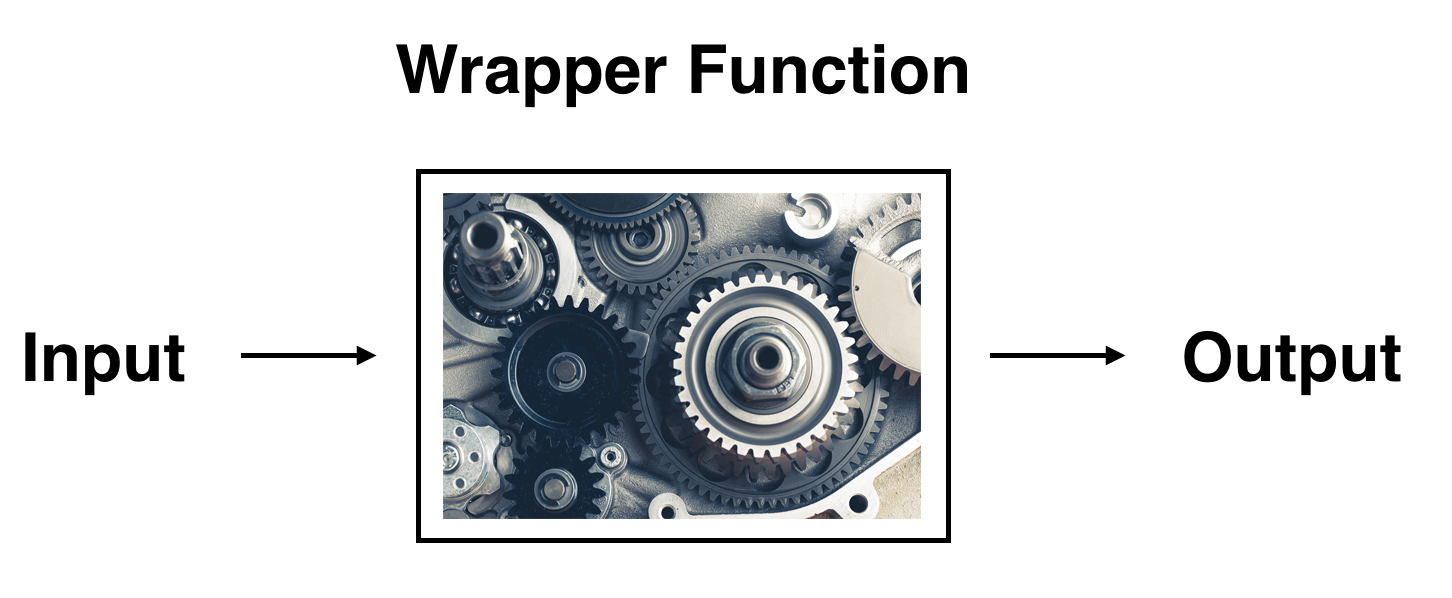FIGURE 5.5: The concept of a wrapper function.

So all you need to worry about is what the inputs look like and what the outputs look like; you leave all the other details “under the hood of the car.” In our regression modeling example, the get_regression_table() function takes a saved lm() linear regression model as input and returns a data frame of the regression table as output. If you’re interested in learning more about the get_regression_table() function’s inner workings, check out Subsection 5.3.3.

Lastly, you might be wondering what the remaining five columns in Table 5.2 are: std_error, statistic, p_value, lower_ci and upper_ci. They are the standard error, test statistic, p-value, lower 95% confidence interval bound, and upper 95% confidence interval bound. They tell us about both the statistical significance and practical significance of our results. This is loosely the “meaningfulness” of our results from a statistical perspective. Let’s put aside these ideas for now and revisit them in Chapter 10 on (statistical) inference for regression. We’ll do this after we’ve had a chance to cover standard errors in Chapter 7, confidence intervals in Chapter 8, and hypothesis testing and $$p$$-values in Chapter 9.

Learning check

(LC5.2) Fit a new simple linear regression using lm(score ~ age, data = evals_ch5) where age is the new explanatory variable $$x$$. Get information about the “best-fitting” line from the regression table by applying the get_regression_table() function. How do the regression results match up with the results from your earlier exploratory data analysis?

### 5.1.3 Observed/fitted values and residuals

We just saw how to get the value of the intercept and the slope of a regression line from the estimate column of a regression table generated by the get_regression_table() function. Now instead say we want information on individual observations. For example, let’s focus on the 21st of the 463 courses in the evals_ch5 data frame in Table 5.3:

TABLE 5.3: Data for the 21st course out of 463
ID score bty_avg age
21 4.9 7.33 31

What is the value $$\widehat{y}$$ on the regression line corresponding to this instructor’s bty_avg “beauty” score of 7.333? In Figure 5.6 we mark three values corresponding to the instructor for this 21st course and give their statistical names:

• Circle: The observed value $$y$$ = 4.9 is this course’s instructor’s actual teaching score.
• Square: The fitted value $$\widehat{y}$$ is the value on the regression line for $$x$$ = bty_avg = 7.333. This value is computed using the intercept and slope in the previous regression table:

$\widehat{y} = b_0 + b_1 \cdot x = 3.88 + 0.067 \cdot 7.333 = 4.369$

• Arrow: The length of this arrow is the residual and is computed by subtracting the fitted value $$\widehat{y}$$ from the observed value $$y$$. The residual can be thought of as a model’s error or “lack of fit” for a particular observation. In the case of this course’s instructor, it is $$y - \widehat{y}$$ = 4.9 - 4.369 = 0.531.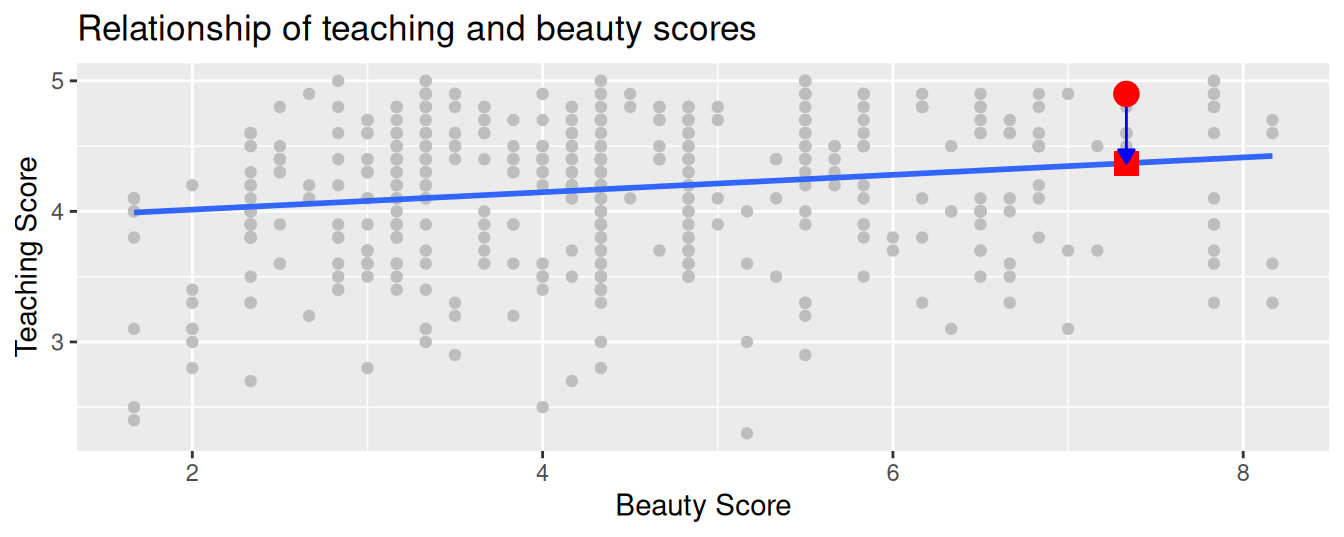FIGURE 5.6: Example of observed value, fitted value, and residual.

Now say we want to compute both the fitted value $$\widehat{y} = b_0 + b_1 \cdot x$$ and the residual $$y - \widehat{y}$$ for all 463 courses in the study. Recall that each course corresponds to one of the 463 rows in the evals_ch5 data frame and also one of the 463 points in the regression plot in Figure 5.6.

We could repeat the previous calculations we performed by hand 463 times, but that would be tedious and time consuming. Instead, let’s do this using a computer with the get_regression_points() function. Just like the get_regression_table() function, the get_regression_points() function is a “wrapper” function. However, this function returns a different output. Let’s apply the get_regression_points() function to score_model, which is where we saved our lm() model in the previous section. In Table 5.4 we present the results of only the 21st through 24th courses for brevity’s sake.

regression_points <- get_regression_points(score_model)
regression_points
TABLE 5.4: Regression points (for only the 21st through 24th courses)
ID score bty_avg score_hat residual
21 4.9 7.33 4.37 0.531
22 4.6 7.33 4.37 0.231
23 4.5 7.33 4.37 0.131
24 4.4 5.50 4.25 0.153

Let’s inspect the individual columns and match them with the elements of Figure 5.6:

• The score column represents the observed outcome variable $$y$$. This is the y-position of the 463 black points.
• The bty_avg column represents the values of the explanatory variable $$x$$. This is the x-position of the 463 black points.
• The score_hat column represents the fitted values $$\widehat{y}$$. This is the corresponding value on the regression line for the 463 $$x$$ values.
• The residual column represents the residuals $$y - \widehat{y}$$. This is the 463 vertical distances between the 463 black points and the regression line.

Just as we did for the instructor of the 21st course in the evals_ch5 dataset (in the first row of the table), let’s repeat the calculations for the instructor of the 24th course (in the fourth row of Table 5.4):

• score = 4.4 is the observed teaching score $$y$$ for this course’s instructor.
• bty_avg = 5.50 is the value of the explanatory variable bty_avg $$x$$ for this course’s instructor.
• score_hat = 4.25 = 3.88 + 0.067 $$\cdot$$ 5.50 is the fitted value $$\widehat{y}$$ on the regression line for this course’s instructor.
• residual = 0.153 = 4.4 - 4.25 is the value of the residual for this instructor. In other words, the model’s fitted value was off by 0.153 teaching score units for this course’s instructor.

At this point, you can skip ahead if you like to Subsection 5.3.2 to learn about the processes behind what makes “best-fitting” regression lines. As a primer, a “best-fitting” line refers to the line that minimizes the sum of squared residuals out of all possible lines we can draw through the points. In Section 5.2, we’ll discuss another common scenario of having a categorical explanatory variable and a numerical outcome variable.

Learning check

(LC5.3) Generate a data frame of the residuals of the model where you used age as the explanatory $$x$$ variable.